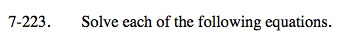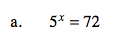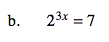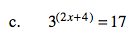Home > A2C > Chapter 7 > Lesson 7.3.5 > Problem7-223

7-223.
1. Solve each of the following equations. Homework Help ✎

1. 5x = 72

2. 23x = 7

3. 3(2x+4) = 17Since x is in the exponent, take the log of both sides and apply the product property.x ≈ 2.657$\log(2)^{3x} = \log(7)$

$3x \cdot \log(2) = \log(7)$

$3x = \frac{\log (7)}{ \log (2)}$

Use your calculator and continue solving the equation.x ≈ −0.711